EXERCISE 7.1

1). Write the fraction representing the shaded portion.
i)ii)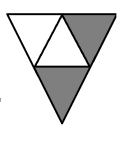iii)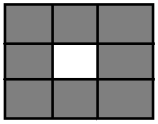iv)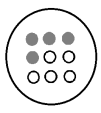v)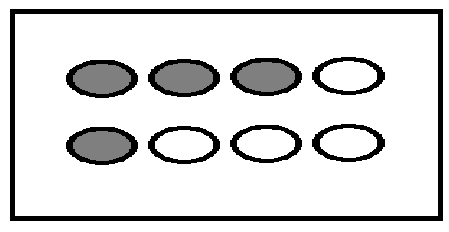vi)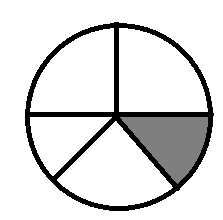vii)viii)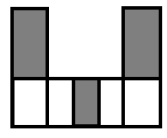ix)x)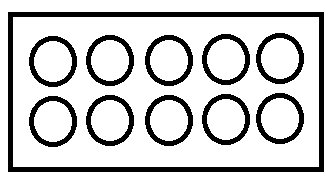xi)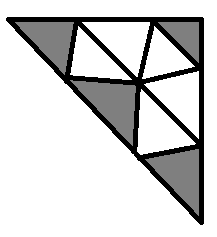Ans:
(i).The hexagon is divided into two equal parts .And  one part is shaded  out of two parts. So it is ( 1/2)
(ii).The triangle is divided into four equal parts.And two parts are shaded out of four parts.So the fraction is ( 2/4).
(iii). The Square is divided into nine equal parts. And eight parts is shaded out of nine parts. so the fraction is (1/9)
(iv). The Circle is divided into eight parts. And four parts are shaded out of eight parts. So the fraction is (4/9)
(v). The rectangle is divided into eight parts. And four parts are shaded out of eight parts. so the fraction is (4/8 )
(vi). The circle is divided into five parts. And one part is shaded out of five parts. so the fraction is (1/5)
(vii). The Circle is divided into four parts. And one part is shaded out of four parts. so the fraction is (1/4)
(viii). The figure is divided into seven parts. And three part are shaded out of seven. so the fraction is (3/7)
(ix). The Square is divided into twelve parts. And three parts are shaded out of twelve. so the fraction is (3/( 12)
(x). The rectangle is divided into ten parts. And no part is shaded out of ten. so the fraction is (0/10)
(xi). The triangle is divided into nine parts. And four parts are shaded out of nine. so the fraction is (4/9)

2).Color the part according to the fraction given.
[i](1/6)                     [ii]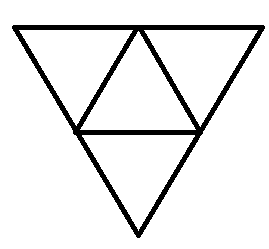(2/4)                        [iii]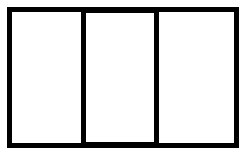(1/3)      iv]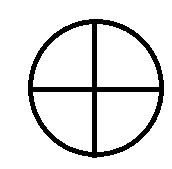(3/4)                [v]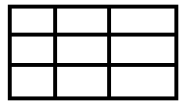(4/9)
[vi]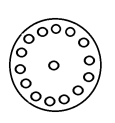(1/4)

3). Identify the error, if any
[i]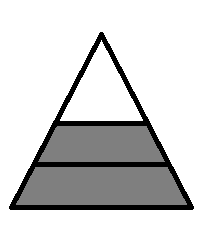This is (1/2)     [ii]This is (1/4)      [iii]This is (3/4)
Ans.
[i] since the portion does not have equal levels.So it is not (1/2)

[ii] since the parts are not equal.So it is not (1/4)
[iii] since the parts are not equal.So it is not (3/4)

4). What fraction of a day is a 8 hours?
Ans. There are 24 hours in a day we have 8 hours.So required portion is (8/24)

5). What fraction of an hour is 40 minutes?
Ans. An hour has 60 minutes and we have 40 minutes.So required portion is (40/60)

6). Arya, Abhimanyu and Vivek share lunch. Arya brought two sandwiches, one made of vegetables and one of jam. The other two boys forgot to bring their lunch. Arya agreed to share his sandwiches so that each person will have equal share of each sandwich
a) How can Arya divide his sandwiches so that each person has an equal share?
Ans. Arya can divide the sandwich into three equal parts i.e. each person gets (1/3)
b). What part of a sandwich will each boy receive?
Ans. Each boy will receive (1/3)

7). Kanchan has three frocks that she wears while playing. The material is good, but the colours are faded. Her mother buys some blue dye and uses it on two of the frocks. What fraction of the Kanchan’s frock did her mother dye?

Ans. Total number of dresses = 3
number of frocks her mother dyes = 2
So the required portion is (2/3)

8). Write the natural numbers from 2 to 12. What fraction of them are prime numbers?
Ans. Natural numbers from 2 to 12 are :2, 3, 4, 5, 6, 7, 8, 9, 10,11, 12.
Total No. of natural numbers given = 11
Number of prime number = 5 [2, 3, 5, 7, 11]
So the required fraction = (5/11)

9). Write the natural numbers from 102 to 113. What fraction of them are prime numbers?
Ans. Natural numbers from 102 to 113 are :
102, 103, 104, 105, 106, 107, 108, 109, 110, 111, 112, 113.
Total number of natural numbers given = 12
Total of prime number = 4 (103, 107, 109, 113)
So the required fraction is (4/12) = (1/3)

10). What fraction of these circles have ‘x’ in them?
(a)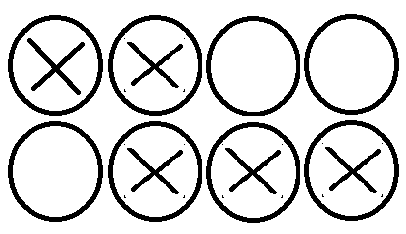(b)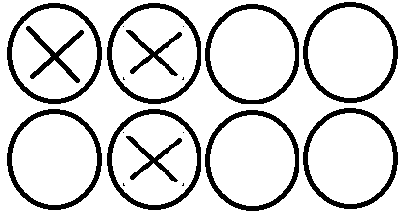(c)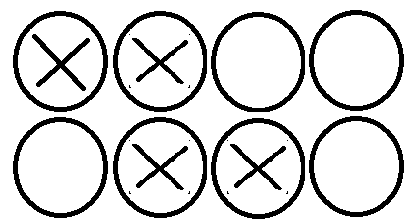Ans: (a)  The total number of circles are eight and five circles are with x’s in them. so the fraction is (5/8)
(b) The total number of circles are eight and three circles are with x ‘s in them. so the fraction is (3/8)
(c) The total number of circles are eight and four circles are with x’s in them. so the fraction is (4/8)

11). Dinesh, Sumith , Ram, Joy, Marshal, Imran, Jayant, Babu, Kabir and Rohan divide to play basketball. The first five boys are in the first team and the rest are in the second team. What fraction of the boys are in the first team?
Ans. Number of boys in the first team = 5
Total number of boys = 10
So the required fraction is (5/10)

12). Kristin received a CD player for her birthday. She has been collecting CDs since then. She brought 3 CDs and received 5 others as gifts. What fraction of her total CDs did she buy?
Ans. Kristin brought CDs =3
Total number of CDs with her = 8
i.e. required portion is (3/8)